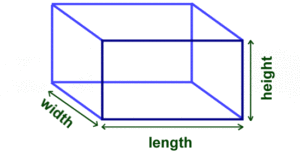# Java: Volume Of Box Program | Java Programs

Java program to find the volume of box –  In this article the java code is used for calculating the the volume of the box. The concepts are vividly explained to make it easier for you to learn.

What is volume?

Volume is a three dimensional space and is denoted by a standard unit of “meter” in physics.  Volume is infact a quantity derived from length. Volume is nothing but measuring how much space a three dimensional object takes up.

If in case it was just a two dimensional object the calculation part would have been a lot simpler.

For a two dimensional object such as a rectangle we could have multiplied the length and the width, but alas that’s only a two dimensional object. For a three dimensional object we have to take in account the “height” too. The height is really the third dimension when it comes to calculating the volume of a box.## Java Volume Of Box Program

A box is a three-dimensional cuboid figure that has its own length, breadth and height. To find the volume of the box, we’ll require the same three dimension values as our input and the resultant output volume is always in cubic units.

For any given problem, to write the code and algorithm, there are 5 basic steps. First is the problem statement, then the constraints, then the inputs followed by outputs and finally the logic or the solution.

Here, our problem statement is, we need to find the volume of a three dimensional box. As we are all well aware, the dimensions of the box can be a whole number as well as fractional, so this is our constraint and hence we make use of the double data type for values.

Our input for this problem would be, values of all the three dimensions- length, breadth and height of double type. The output is, the volume of the box which is also of double type.

Now, coming to the solution of this problem, it is always more convenient to read inputs at runtime than giving it directly in the code. Hence, we make use of the Scanner class in Java that allows us to do so. This is one of the system input methods.

Therefore, we take the input length, breadth and height by first initializing the class by creating object of Scanner class and making use of nextDouble() method of the Scanner class as follows:

After gathering all the required output, we need to calculate the volume of the box. As mentioned, since the box is of cuboid shape here, we are all well aware that,

volume of cuboid= length * breadth * height

Making use of the same formula, we calculate the volume and save it in another variable like below:

double volume= l*b*h;

This variable volume is nothing but, our resultant output which can then be displayed in out output console screen.

Output:

x

## Plus Star Pattern Java Program | Patterns

Java program to print plus star pattern program – We have written the below print/draw ...